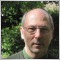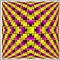# How to initialize a double to the max value ? double VarMax = ???;395

Hello,

How to initialize a double to the max value ?

double VarMax = ???;

Thanks,

Pierre8r2397

Max value of a double in mql4 is documented as: 1.7 * e308 not sure how you code that though.

Edit: I thought about that some more I think you code it like this ...

`double VarMax = 1.7 * 10^308;`1863

double type is 8 bytes in size, if you do this

```double VarMax =MathPow (2, 1024) ;

you will get

`1.#INF`

- LOL - what am I talking about ?, that's not even the maximum double value !

:D

Ref :

https://en.wikipedia.org/wiki/Double-precision_floating-point_format2397

my version doesnt work, if you Print() to get the value of:
`double VarMax = 1.7 * 10^308;`
it is 540.6 LOL1863

Man, that delete link is surely handy for me ;D2397

I think ^ didnt work the way I thought it would ... so instead, this might be it:

`   double VarMax = 1.7 * (MathPow(10,308);`2397

onewithzachy:

Man, that delete link is surely handy for me ;D

yeah i deleted my earlier post too because I realized it wasnt making sense lol I edited the one after that so the thread at least has some continuity :D1863

SDC:

yeah i deleted my earlier post because I realized it wasnt making sense lol I edited the one after that so the thread at least has some continuity :D

I just ... accidentally delete mine :D

SDC:

I think ^ didnt work the way I thought it would ... so instead, this might be it:

` double VarMax = 1.7 * (MathPow(10,308);`

I think you're right, (https://docs.mql4.com/basis/types/double) or to be a little more precisely

`double VarMax = 1.7976931348623158 * (MathPow(10,308);`

base on MQL5 doc.

:D2397

Yes it appears the mql4 docs dont give a precise value for the base of that calculation so your version should be the correct max value

Edit: having said that the actual biggest double is a little larger than that because the base value 1.7976931348623158 is rounded down to 16dp395

Hello,

Dont you think such a constant is missing into the MQL4 language ?

Pierre8r

```//+------------------------------------------------------------------+
//|                                           TestDoubleValueMax.mq4 |
//|                                                                  |
//+------------------------------------------------------------------+

//+------------------------------------------------------------------+
//| script program start function                                    |
//+------------------------------------------------------------------+
int start()
{
//----
double VarMax = 1.7 * (MathPow(10,308));

//----
return(0);
}
//+------------------------------------------------------------------+```20207

tintin92:
How to initialize a double to the max value ?
I use this:
`#define INF 0x6FFFFFFF // Not quite infinite, Jul 2029, or 1,879,048,191`
It's not infinite but larger than you'll find in FX and it can be used in signed computations.